# Functions As Maps

Go back to  'Functions'

Learn from the best math teachers and top your exams

• Live one on one classroom and doubt clearing
• Practice worksheets in and after class for conceptual clarity
• Personalized curriculum to keep up with school

Maps are a convenient way to visualise functions, or more generally, the association between two sets. A map relates one set to another using some rule. For example, suppose the rule is, $$y = f(x) = {x^2},\;{\rm{Set\,A}} = \{ 1,\;2,\;3,\;4\} ,\;{\rm{Set\,B}} = \{ 1,\;4,\;9,\;16\}$$

We can show this as a map.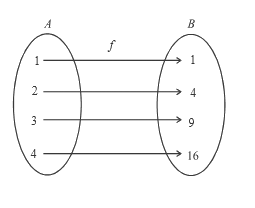The elements in $${\text{set B}}$$ are the images of the elements of $${\text{set A}}$$ under the action of $$f.$$

$${\text{Set A}}$$ would be called the domain of $$f\left\{ {{\text{the set of all input values}}}\right\},$$  and $${\text{set B}}$$ the co-domain of

$$f\left\{ {{\text{the set in which the output values lie}}}\right\}.$$ Here, the co-domain is the same as the range{precisely

the set of all outputs}, but in general, range would be a subset of the co-domain

Range $$\subseteq$$ co-domain.

For our purposes, co-domain is not of much significance. It is more of a matter of convenience to specify the co-domain.

Suppose I want to talk about $$f(x) = \sqrt {a - x} + \sqrt {x - b} ,\,a > b > 0$$ (the function we analysed earlier). Note that the domain is $$[b,\,a].$$ The output will obviously be real and positive (This much I can tell just by looking at the definition of $$f(x)$$).

Hence, I could specify the definition of $$f$$ as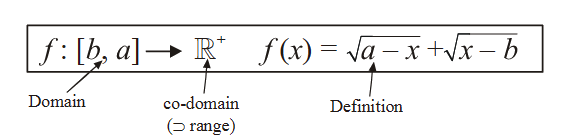As we can see above, co-domain just tells us that all the outputs will lie in it. The exact set of outputs (the range) will lie in the co-domain, and will be its subset.

We know that the range for this $$f(x)$$ is $$[\sqrt {a - b} ,\sqrt {2(a - b)} ]$$

Therefore, I can also specify $$f$$ as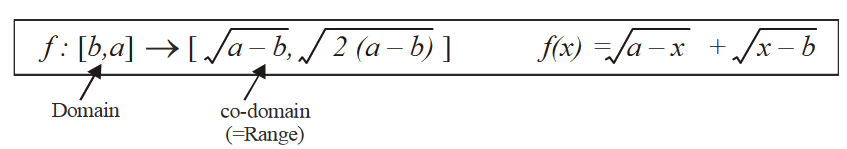In this definition, co-domain is the same as range. Note that co-domain can be at the most equal to range (not smaller).

The map in the figure above is an example of a one-one and onto map. Let us see what this means, and what are the various possible kinds of maps.

(A)  ONE-ONE / MANY – ONE

One-one implies that each element in the domain set is mapped to only one element in the co-domain set, that is, no two inputs map to the same output.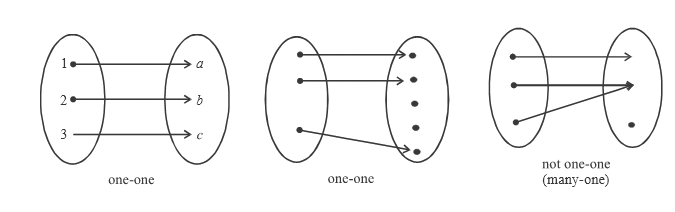The one-one condition can be written mathematically as

$$f({x_1}) = f({x_2})$$  if and only if  $${x_1} = {x_2}$$

This, stated in words, says that two outputs from f can be equal if and only if the  corresponding inputs to f are equal.

If $$f({x_1}) = f({x_2})$$ does not imply $${x_1} = {x_2}$$, then the function is many-one. Therefore, given $$f,$$ we can find whether its one-one or not by solving the equation $$f({x_1}) = f({x_2})$$. If this equation yields $${x_1} = {x_2}$$, then $$f$$ is one-one else not. As an example, consider $$f(x)=x^2.$$ Is this one-one, or many one?

Solving

$$f({x_1}) = f({x_2}) \qquad \Rightarrow \qquad x_1^2 = x_2^2$$

\begin{align}&\Rightarrow \qquad ({x_1} + {x_2})({x_1} - {x_2}) = 0\\& \Rightarrow \qquad {x_1} = {x_2}\,\,\,{\rm{or}}\,\,\,{x_1} = - {x_2} \end{align}

Hence  $$f({x_1}) = f({x_2})$$  does not uniquely imply  $${x_1} = {x_2}$$  (Because another case exists,  $${x_1} = -{x_2}$$ )

For example  $$f(3) = f( - 3) = 9$$ (One output for two different inputs). Hence $$f(x) = {x^2}$$  is many-one

On a graph, this implies that if we draw any horizontal line, and it intersects the graph at the most once, then it is one-one, else it is many-one.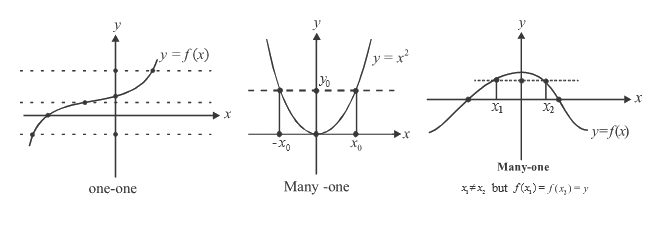Note: One-many or many-many maps also exist but they are not functions. A function implies that each element in the domain is associated with one and only one element in the co-domain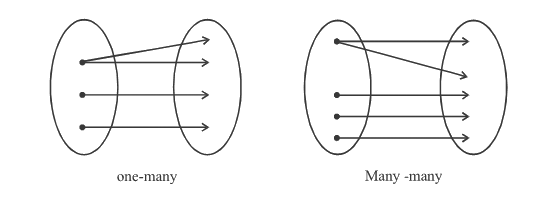For the graph of a valid function, this implies that if we draw a vertical line, it should intersect the graph at the most once. Otherwise, it is not a function.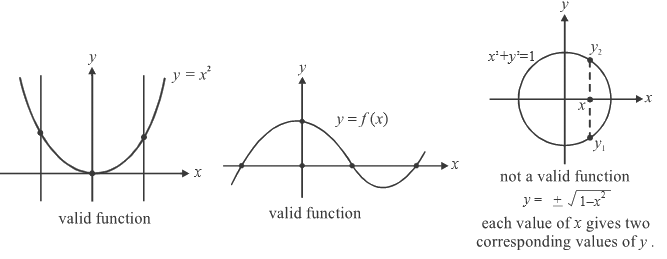(B)  INTO/ONTO

Look carefully at the three maps below. The first and the third are many-one, while the second is one-many.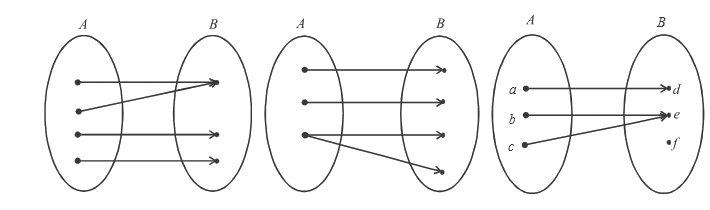Any other differences? For the first two maps, each element in the co-domain ($${\text{Set B}}$$) is ‘covered’, that is, associated with some element in the domain ($${\text{Set A.}}$$) For the third map, one element $$(\{ f\})$$ in the co-domain is ‘left out’ (is not associated with any element in the domain, or in other words, does not have a pre-image). The first two maps are onto while the third is into.

Stating formally, an onto map is a map in which the range (the set of all images of the elements of the domain) ‘covers’ the entire co-domain, that is, $${\bf{\text{Range}}}={\bf{\text{Co-domain}}}.$$

If $${\text{Range}}\subset{\text{Co-domain}}$$ (like the third map above), then it is an into map.

An example will make all this more clear: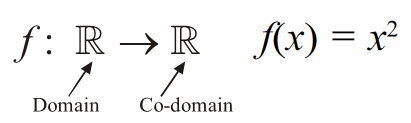The range is the set of all non-negative numbers, as $${x^2} \ge 0.$$ Hence, Range $$\subset$$ Co-domain and the function is into. On the other hand, for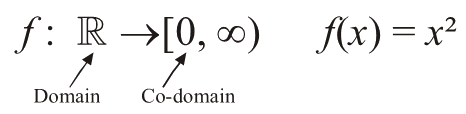Range = Co-domain and the map is onto.

Functions
grade 11 | Questions Set 1
Functions
Functions
grade 11 | Questions Set 2
Functions